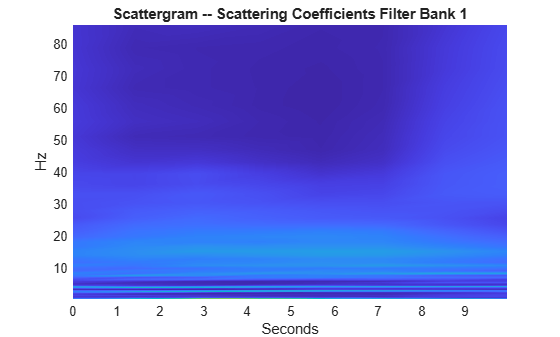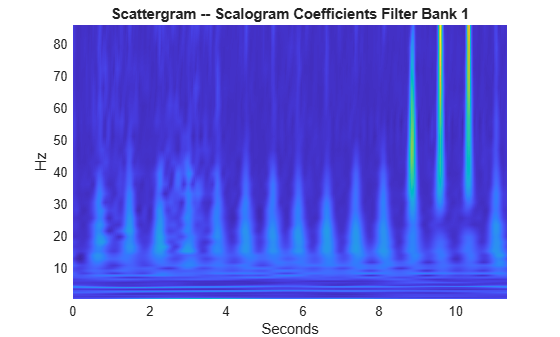# scattergram

Visualize scattering or scalogram coefficients

## Syntax

``img = scattergram(sf,S)``
``img = scattergram(sf,U)``
``img = scattergram(___,Name,Value)``
``scattergram(___)``

## Description

````img = scattergram(sf,S)` returns the scattergram as a matrix for the first-order scattering coefficients, `S`. The matrix `S` is the output of `scatteringTransform` computed using the wavelet time scattering network, `sf`.```
````img = scattergram(sf,U)` returns the scattergram as a matrix for the first-order scalogram coefficients, `U`. The matrix `U` is the output of `scatteringTransform` computed using the wavelet time scattering network, `sf`.```
````img = scattergram(___,Name,Value)` returns the scattergram with additional options specified by one or more `Name,Value` pair arguments. You can use this syntax with any of the input syntaxes shown previously.```

example

````scattergram(___)` with no output arguments plots the scattergram in the current figure. You can use any of the input syntaxes shown previously.```

## Examples

collapse all

Load an ECG signal sampled at 180 Hz. Create a wavelet time scattering network that can be used with the signal.

```load wecg Fs = 180; sf = waveletScattering('SignalLength',numel(wecg),... 'SamplingFrequency',Fs);```

Calculate the scattering transform of the signal.

`[S,U] = scatteringTransform(sf,wecg);`

Visualize the scattergram for the first-order scattering and scalogram coefficients.

`scattergram(sf,S,'FilterBank',1)````figure scattergram(sf,U,'FilterBank',1)```## Input Arguments

collapse all

Wavelet time scattering network, specified as a `waveletScattering` object.

Scattering coefficients, specified as a cell array. `S` is the output of `scatteringTransform` computed using the scattering network, `sf`. For more information, see `scatteringTransform`.

Scalogram coefficients, specified as a cell array. `U` is the output of `scatteringTransform` computed using the scattering network, `sf`. For more information, see `scatteringTransform`.

### Name-Value Arguments

Specify optional pairs of arguments as `Name1=Value1,...,NameN=ValueN`, where `Name` is the argument name and `Value` is the corresponding value. Name-value arguments must appear after other arguments, but the order of the pairs does not matter.

Before R2021a, use commas to separate each name and value, and enclose `Name` in quotes.

Example: `'FilterBank',1` specifies the first filter bank.

Filter bank index, specified as a positive number between 1 and the number of filter banks in `sf` inclusive. `scattergram` returns the scattergram for the specified filter bank in `sf`. The number of filter banks in `sf` is equal to the number of specified `QualityFactors` in `sf`.

If `FilterBank` is greater than 1, `scattergram` averages the scalogram or scattering coefficients over all paths terminating at each wavelet bandpass filter. To obtain paths with a common parent, use the `'Parent'` name-value pair.

Path parent index, specified as a nonnegative integer. The scalar `P` is a nonnegative integer representing the `P`-th wavelet filter at the filter bank `FilterBank` − 1. `scattergram` returns the scattergram for the path at the specified filter bank with parent `P`. If `FilterBank` is equal to 1, the zeroth filter bank corresponds to the input signal in the case of the scalogram coefficients and the lowpass filtering of the input signal with the scaling function in the case of the scattering coefficients. Lower values of `P` correspond to wavelets with higher bandpass frequencies.

If you specify `P`, you must specify the `FilterBank` name-value pair.

If you specify a value for `P` which results in a single child, the output `img` is a vector. The scattergram of a single child is a line plot. If you specify a value for `P` that results in no children, `scattergram` returns the scattergram for the filter bank specified by `FilterBank`.

## Output Arguments

collapse all

Scattergram, returned as a real-valued matrix or vector. If you use the `Parent` name-value pair and specify a value which results in a single child, `img` is a vector. If the parent has more than one child, `img` is a matrix.

## Version History

Introduced in R2018b